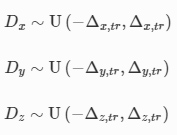## Content Provided By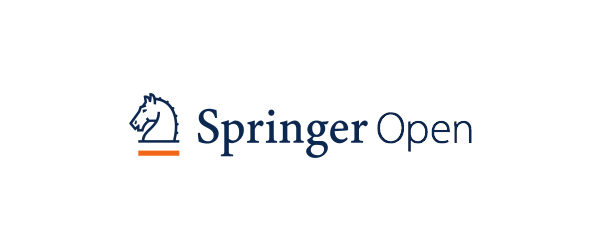## Apparatus used

Bijie Bai, Yi Luo, Tianyi Gan, Jingtian Hu, Yuhang Li, Yifan Zhao, Deniz Mengu, Mona Jarrahi & Aydogan Ozcan

## Introduction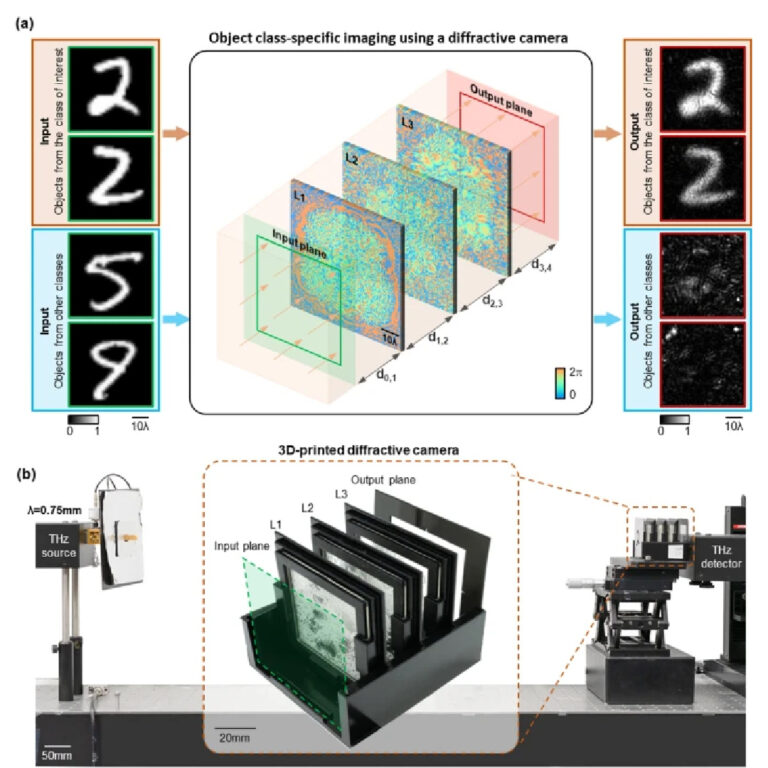## Class-specific imaging using diffractive cameras We first numerically demonstrate the class-specific camera design using the MNIST handwritten digit dataset, to selectively image handwritten digit ‘2’ (the object class of interest) while instantaneously erasing the other handwritten digits. As illustrated in Fig. 2a, a three-layer diffractive imager with phase-only modulation layers was trained under an illumination wavelength of λ. Each diffractive layer contains 120 × 120 trainable transmission phase coefficients (i.e., diffractive features/neurons), each with a size of ~ 0.53λ. The axial distance between the input/sample plane and the first diffractive layer, between any two consecutive diffractive layers, and between the last diffractive layer and the output plane were all set to ~ 26.7λ. The phase modulation values of the diffractive neurons at each transmissive layer were iteratively updated using a stochastic gradient-descent-based algorithm to minimize a customized loss function, enabling object class-specific imaging. For the data class of interest, the training loss terms included the normalized mean square error (NMSE) and the negative Pearson Correlation Coefficient (PCC)  between the output image and the input, aiming to optimize the image fidelity at the output plane for the correct class of objects. For all the other classes of objects (to be all-optically erased), we penalized the statistical similarity between the output image and the input object (see “Methods” section for details). This well-balanced training loss function enabled the output images from the non-target classes of objects (i.e., the handwritten digits 0, 1, 3–9) to be all-optically erased at the output FOV, forming speckle-like background patterns with lower average intensity, whereas all the input objects of the target data class (i.e., handwritten examples of digit 2) formed high-quality images at the output plane. The resulting diffractive layers that are learned through this data-driven training process are reported in Fig. 2b, which collectively function as a spatial mode filter that is data class-specific.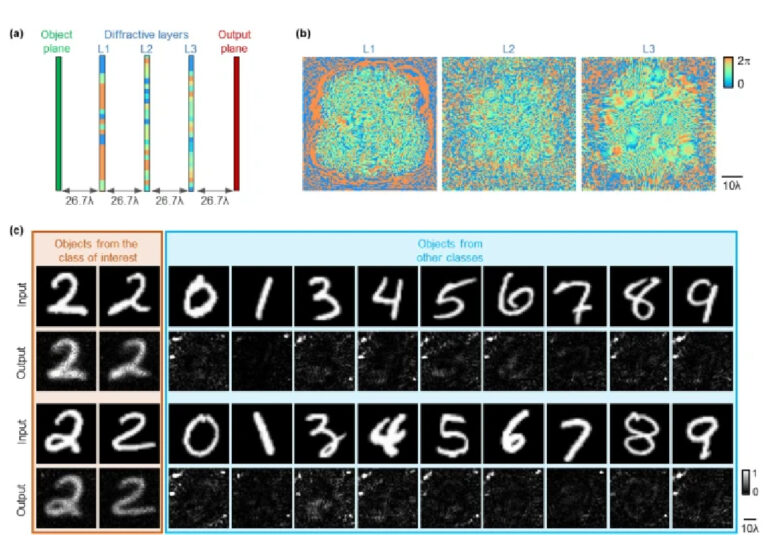## Design schematic and blind testing results of the class-specific diffractive camera. a The physical layout of the three-layer diffractive camera design. b Phase modulation patterns of the converged diffractive layers of the camera. c The blind testing results of the diffractive camera. The output images were normalized using the same constant for visualization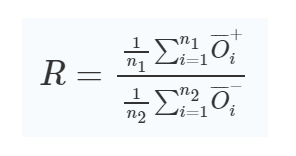## We also quantified the blind testing performance of each diffractive camera design by calculating the average PCC value between the output images and the ground truth (i.e., input objects); see Fig. 3b. For this quantitative analysis, the MNIST testing dataset was first divided into target class objects (n1= 1032 handwritten test objects for digit ‘2’) and non-target class objects (n2= 8968 handwritten test objects for all the other digits), and the average PCC value was calculated separately for each object group. For the target data class of interest, the higher PCC value presents an improved imaging fidelity. For the other, non-target data classes, however, the absolute PCC values were used as an “erasure figure-of-merit”, as the PCC values close to either 1 or −1 can indicate interpretable image information, which is undesirable for object erasure. Therefore, the average PCC values of the target class objects (n1) and the average absolute PCC values of the non-target classes of objects (n2) are presented in the first two charts in Fig. 3b. The depth advantage of the class-specific diffractive camera designs is clearly demonstrated in these results, where a deeper diffractive imager with e.g., five transmissive layers achieved (1) a better output image fidelity and a higher average PCC value for imaging the target class of objects, and (2) an improved all-optical erasure of the undesired objects (with a lower absolute PCC value) for the non-target data classes as shown in Fig. 3b.In addition to these, a deeper diffractive camera also creates a stronger signal intensity separation between the output images of the target and non-target data classes. To quantify this signal-to-noise ratio advantage at the output FOV, we defined the average output intensity ratio (R) of the target to non-target data classes as: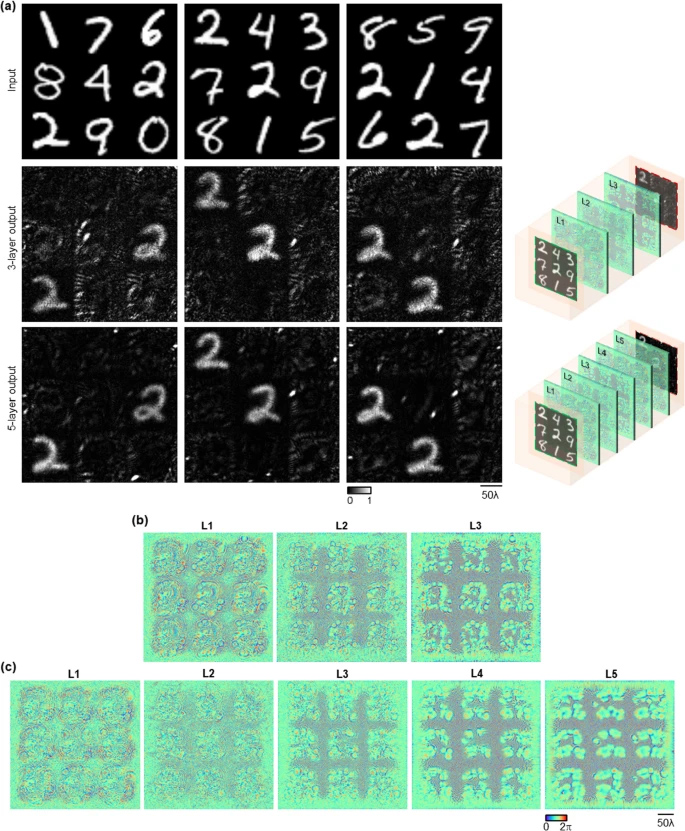## where the numerator is the average output intensity of n1= 1032 test objects from the target data class (denoted as O+i), and the denominator is the average output intensity of n2= 8968 test objects from all the other data classes (denoted as O−i). The R values of three-, four-, and five-layer diffractive camera designs were found to be 1.354, 1.464, and 1.532, respectively, as summarized in Fig. 3b. These quantitative results once again confirm that a deeper diffractive camera with more trainable layers exhibits a better performance in its class-specific imaging task and achieves an improved signal-to-noise ratio at its output. Note that, a class-specific diffractive camera trained with the standard grayscale MNIST images retains its designed functionality even when the input objects face varying illumination conditions. To demonstrate this, we first blindly tested the five-layer diffractive camera design reported in Fig. 3a under varying levels of intensity (from low to high intensity and eventually saturated, where the grayscale features of the input objects became binary). As reported in Additional file 2: Movie S1, the diffractive camera selectively images the input objects from the target class and robustly erases the information of the non-target classes of input objects, regardless of the intensity, even when the objects became saturated and structurally deformed from their grayscale features. We further blindly tested the same five-layer diffractive camera design reported in Fig. 3a with the input objects illuminated under spatially non-uniform intensity distributions, deviating from the training features. As shown in Additional file 3: Movie S2, the class-specific diffractive camera still worked as designed under non-uniform input illumination intensities, demonstrating its effectiveness and robustness in handling complex scenarios with varying lighting conditions. These input distortions highlighted in Additional file 2: Movie S1, Additional file 3: Movie S2 were never seen/used during the training phase, and illustrate the generalization performance of our diffractive camera design as an optical mode filter, performing class-specific imaging. Simultaneous imaging of multiple objects from different data classesIn a more general scenario, multiple objects of different classes can be presented in the same input FOV. To exemplify such an imaging scenario, the input FOV of the diffractive camera was divided into 3 × 3 subregions, and a random handwritten digit/object could appear in each subregion (see e.g., Fig. 4). Based on this larger FOV with multiple input objects, a three-layer and a five-layer diffractive camera were separately designed to selectively image the whole input plane, all-optically erasing all the presented objects from the non-target data classes (Fig. 4a). The design parameters of these diffractive cameras were the same as the cameras reported in the previous subsection, except that each diffractive layer was expanded from 120 × 120 to 300 × 300 diffractive pixels to accommodate the increased input FOV. During the training phase, 48,000 MNIST handwritten digits appeared randomly at each subregion, and the handwritten digit ‘2’ was selected as our target data class to be specifically imaged. The diffractive layers of the converged camera designs are shown in Fig. 4b for the three-layer diffractive camera and in Fig. 4c for the five-layer diffractive camera.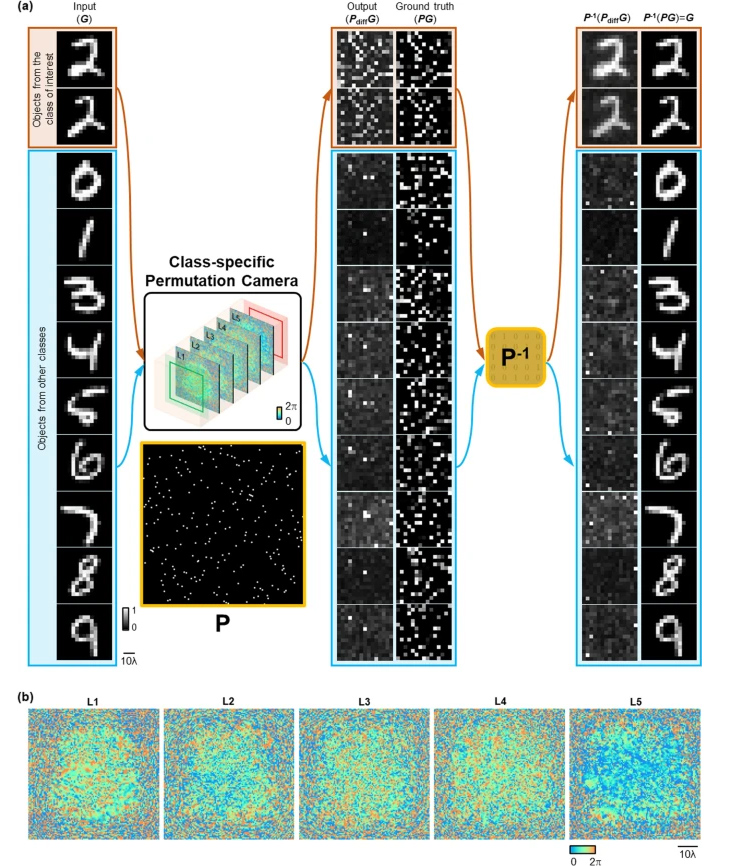## During the blind testing phase of each of these diffractive cameras, the input test objects were randomly generated using the combinations of 10,000 MNIST test digits (not included in the training). Our imaging results reported in Fig. 4a reveal that these diffractive camera designs can selectively image the handwritten test objects from the target data class, while all-optically erasing the other objects from the remaining digits in the same FOV, regardless of which subregion they are located at. It is also demonstrated that, compared with the three-layer design, the deeper diffractive camera with five trained layers generated output images with improved fidelity and higher contrast for the target class of objects, as shown in Fig. 4a. At the same time, this deeper diffractive camera achieved stronger suppression of the objects from the non-target data classes, generating lower output intensities for these undesired objects. Class-specific camera design with random object displacements over a large input field-of-view In consideration of different imaging scenarios, where the target objects can appear at arbitrary spatial locations within a large input FOV, we further demonstrated a class-specific camera design that selectively images the input objects from a given data class within a large FOV. As the space-bandwidth product at the input (SBPi) and the output (SBPo) planes increased in this case, we used a deeper architecture with more diffractive neurons, since in general the number of trainable diffractive features in a given design needs to scale proportional to SBPi × SBPo [39, 40]. Therefore, we used seven diffractive layers, each with 300 × 300 diffractive neurons/pixels. During the training phase, 48,000 MNIST handwritten digits were randomly placed within the input FOV of the camera, one by one, and the handwritten digit ‘2’ was selected to be specifically imaged at the corresponding location on the output/image plane while the input objects from the other classes were to be erased (see the “Methods” section). As demonstrated in Additional file 4: Movie S3, test objects from the target data class (the handwritten digit ‘2’) can be faithfully imaged regardless of their varying locations, while the objects from the other data classes were all-optically erased, only yielding noisy images at the output plane. This deeper diffractive camera exhibits class-specific imaging over a larger input FOV regardless of the random displacements of the input objects. The blind testing performance shown in Additional file 4: Movie S3 can be further improved with wider and deeper diffractive camera architectures with more trainable features to better cope with the increased space-bandwidth product at the input and output fields-of-view.Class-specific permutation camera design Apart from directly imaging the objects from a target data class, a class-specific diffractive camera can also be designed to output pixel-wise permuted images of target objects, while all-optically erasing other types of objects. To demonstrate this class-specific image permutation as a form of all-optical encryption, we designed a five-layer diffractive permutation camera, which takes MNIST handwritten digits as its input and performs an all-optical permutation only on the target data class (e.g., handwritten digit ‘2’). The corresponding inverse permutation operation can be sequentially applied on the pixel-wise permuted output images to recover the original handwritten digits, ‘2’. The other handwritten digits, however, will be all-optically erased, with noise-like features appearing at the output FOV, before and after the inverse permutation operation (Fig. 5a). Stated differently, the all-optical permutation of this diffractive camera operates on a specific data class, whereas the rest of the objects from other data classes are irreversibly lost/erased at the output FOV.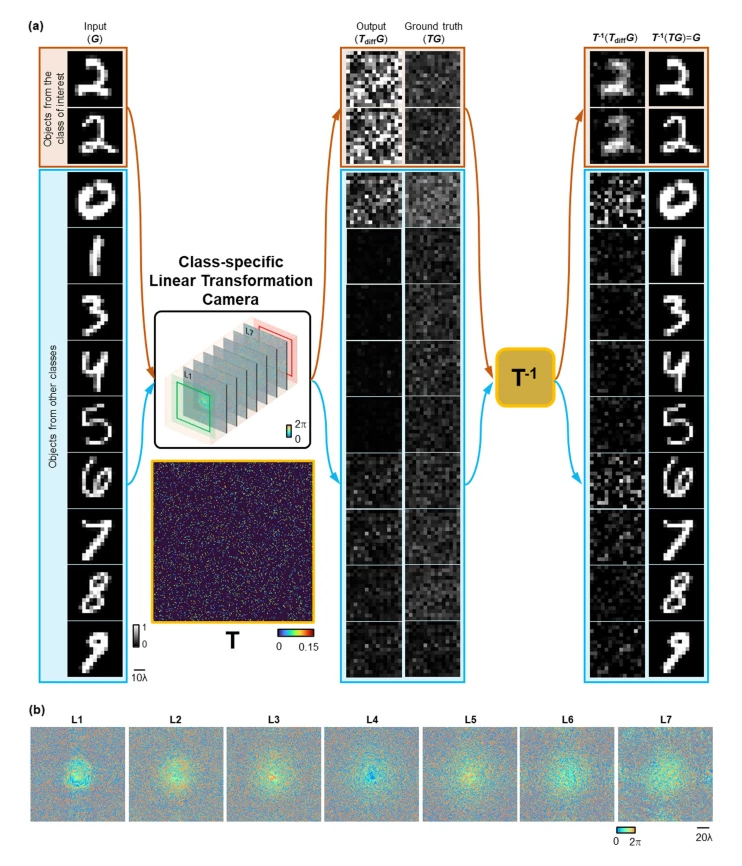## Class-specific linear transformation camera. a Illustration of a seven-layer diffractive camera trained to perform a class-specific linear transformation (denoted as TT) with instantaneous all-optical erasure of the other classes of objects at its output FOV. This class-specific all-optical linear transformation operation performed by the diffractive camera results in uninterpretable patterns of the target objects at the output FOV, which cannot be decoded without the knowledge of the transformation matrix, TT, or its inverse. By applying the inverse linear transformation (TT−1) on the output images of the diffractive camera, the original images of interest from the target data class can be faithfully reconstructed. On the other hand, the input objects from the other data classes end up in noise-like patterns both before and after applying the inverse linear transformation, demonstrating the success of this class-specific linear transformation camera design. The output images were normalized using the same constant for visualization. b Phase modulation patterns of the converged diffractive layers of the class-specific linear transformation cameraExperimental demonstration of a class-specific diffractive camera We experimentally demonstrated the proof of concept of a class-specific diffractive camera by fabricating and assembling the diffractive layers using a 3D printer and testing it with a continuous wave source at λ= 0.75 mm (Fig. 7a). For these experiments, we trained a three-layer diffractive camera design using the same configuration as the system reported in Fig. 2, with the following changes: (1) the diffractive camera was “vaccinated” during its training phase against potential experimental misalignments , by introducing random displacements to the diffractive layers during the iterative training and optimization process (Fig. 7b, see the “Methods” section for details); (2) the handwritten MNIST objects were down-sampled to 15 × 15 pixels to form the 3D-fabricated input objects; (3) an additional image contrast-related penalty term was added to the training loss function to enhance the contrast of the output images from the target data class, which further improved the signal-to-noise ratio of the diffractive camera design. The resulting diffractive layers, including the pictures of the 3D-printed camera, are shown in Fig. 7b, c.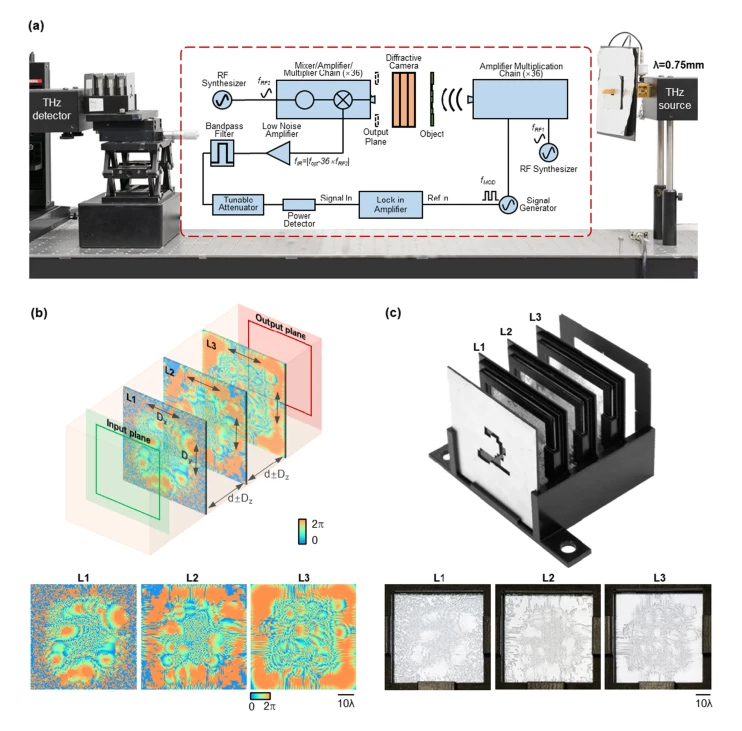## Experimental setup for object class-specific imaging using a diffractive camera. a Schematic of the experimental setup using THz illumination. b Schematic of the misalignment resilient training of the diffractive camera and the converged phase patterns. c Photographs of the 3D printed and assembled diffractive system To blindly test the 3D-assembled diffractive camera (Fig. 7c), 12 different MNIST handwritten digits, including three digits from the target data class (digit ‘2’) and nine digits from the other data classes were used as the input test objects of the diffractive camera. The output FOV of the diffractive camera (36 × 36 mm2) was scanned using a THz detector forming the output images. The experimental imaging results of our 3D-printed diffractive camera are demonstrated in Fig. 8, together with the input test objects and the corresponding numerical simulation results for each input object. The experimental results show a high degree of agreement with the numerically expected results based on the optical forward model of our diffractive camera, and we observe that the test objects from the target data class were imaged well, while the other non-target test objects were completely erased at the output FOV of the camera. The success of these proof-of-concept experimental results further confirms the feasibility of our class-specific diffractive camera design.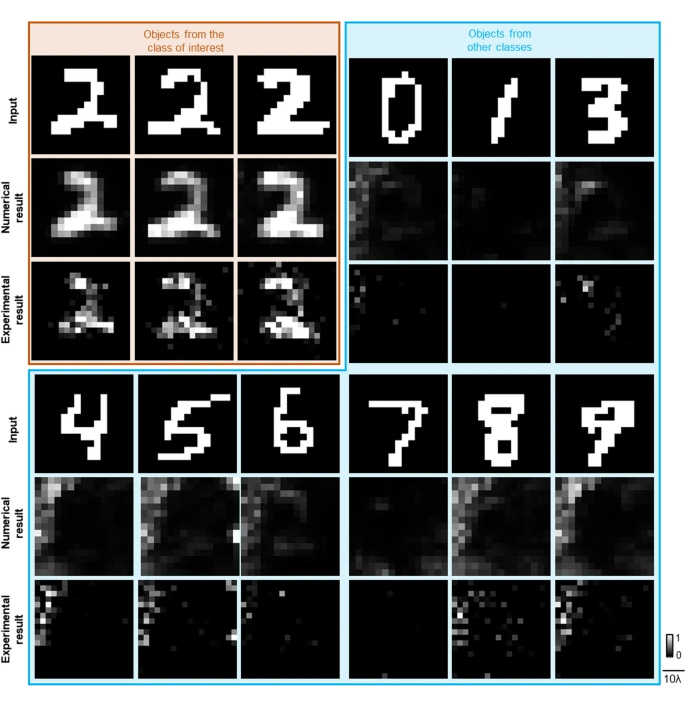## Forward-propagation model of a diffractive camera For a diffractive camera with N diffractive layers, the forward propagation of the optical field can be modeled as a sequence of (1) free-space propagation between the lth and (l + 1)th layers (l=0,1,2,…,N), and (2) the modulation of the optical field by the lth diffractive layer (l=1,2,…,N), where the 0th layer denotes the input/object plane and the (N + 1)th layer denotes the output/image plane. The free-space propagation of the complex field is modeled following the angular spectrum approach . The optical field ul(x,y) right after the lth layer after being propagated for a distance of d can be written as :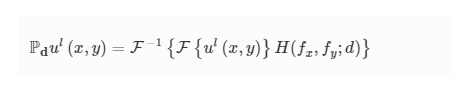## where Pd represents the free-space propagation operator, F and F−1 are the two-dimensional Fourier transform and the inverse Fourier transform operations, and H(fx,fy;d) is the transfer function of free space: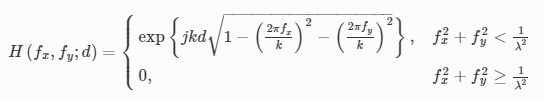## where j=−1−−−√$j=\sqrt{-1}$, k=2πλ$k=\frac{2\pi }{\lambda }$ and λ$\lambda$ is the wavelength of the illumination light. fx${f}_{x}$ and fy${f}_{y}$ are the spatial frequencies along the x$x$ and y$y$ directions, respectively.We consider only the phase modulation of the transmitted field at each layer, where the transmittance coefficient tl${t}^{l}$of thelth diffractive layer can be written as: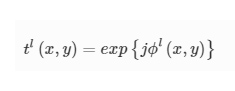## where ϕl(x,y) denotes the phase modulation of the trainable diffractive neuron located at (x,y) position of the lth diffractive layer. Based on these definitions, the complex optical field at the output plane of a diffractive camera can be expressed as: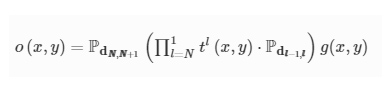## Training loss functionThe reported diffractive camera systems were optimized by minimizing the loss functions that were calculated using the intensities of the input and output images. The input and output intensities G and O, respectively, can be written as: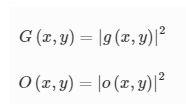## The loss function, calculated using a batch of training input objects GG with the corresponding output images OO can be defined as: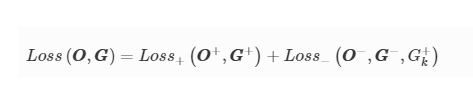## where OO+,GG+ represent the output and input images from the target data class (i.e., desired object class), and OO−,GG− represent the output and input images from the other data classes (to be all-optically erased), respectively. The Loss+ is designed to reduce the NMSE and enhance the correlation between any target class input object O+ and its output image G+, so that the diffractive camera learns to faithfully reconstruct the objects from the target data class, i.e.,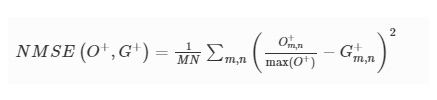## where α1 and α2 are constants and NMSE is defined as: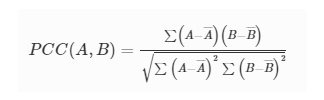## m$m$ and n$n$ are the pixel indices of the images, and MN$MN$ represents the total number of pixels in each image. The output image O+${O}^{+}$ was normalized by its maximum pixel value, max(O+)$\mathrm{m}\mathrm{a}\mathrm{x}\left({O}^{+}\right)$. The PCC value between any two images A$A$ and B$B$ is calculated using :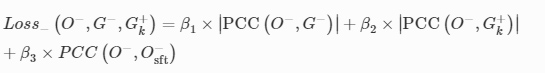## The term (1−PCC(O+,G+)) was used in Loss+ in order to maximize the correlation between O+ and G+, as well as to ensure a non-negative loss value since the PCC value of any two images is always between − 1 and 1. Different fromLoss+, the Loss− function is designed to reduce (1) the absolute correlation between the output O− and its corresponding input G−, (2) the absolute correlation between O− and an arbitrary object G+k from the target class, and (3) the correlation between O− and itself shifted by a few pixels O−sft, which can be formulated as: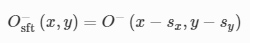## where β1, β2 and β3 are constants. Here the G+k refers to an image of an object from the target data class in the training set, which was randomly selected for every training batch, and the subscript k refers to a random index. In other words, within each training batch, the PCC(O−,G+k) was calculated using the output image from the non-target data class and a random ground truth image from the target class. By adding such a loss term, we prevent the diffractive camera from converging to a solution where all the output images look like the target object. The O−sft was obtained using: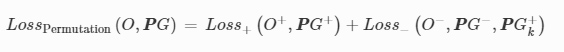## where sx=sy=5 denote the number of pixels that O− is shifted in each direction. Intuitively, a natural image will maintain a high correlation with itself, shifted by a small amount, while an image of random noise will not. By minimizing PCC(O−,O−sft), we forced the diffractive camera to generate uninterpretable noise-like output patterns for input objects that do not belong to the target data class. The coefficients (α1,α2,β1,β2,β3) in the two loss functions were empirically set to (1, 3, 6, 3, 2).Digital implementation and training scheme The diffractive camera models reported in this work were trained with the standard MNIST handwritten digit dataset under λ=0.75mm illumination. Each diffractive layer has a pixel/neuron size of 0.4 mm, which only modulates the phase of the transmitted optical field. The axial distance between the input plane and the first diffractive layer, the distances between any two successive diffractive layers, and the distance between the last diffractive layer and the output plane are set to 20 mm, i.e., dl−1,l=20mm(l=1,2,…,N+1). For the diffractive camera models that take a single MNIST image as its input (e.g., reported in Figs. 2, 3), each diffractive layer contains 120 × 120 diffractive pixels. During the training, each 28 × 28 MNIST raw image was first linearly upscaled to 90 × 90 pixels. Next, the upscaled training dataset was augmented with random image transformations, including a random rotation by an angle within [−10∘,+10∘], a random scaling by a factor within [0.9, 1.1], and a random shift in each lateral direction by an amount of [−2.13λ,+2.13λ]. For the diffractive camera model reported in Fig. 4 that takes multiplexed objects as its input, each diffractive layer contains 300 × 300 diffractive pixels. The MNIST training digits were first upscaled to 90 × 90 pixels and then randomly transformed with [−10∘,+10∘] angular rotation, [0.9, 1.1] scaling, and [−2.13λ,+2.13λ] translation. Nine different handwritten digits were randomly selected and arranged into 3 × 3 grids, generating a multiplexed input image with 270 × 270 pixels for the diffractive camera training. For the diffractive permutation camera reported in Fig. 5, each diffractive layer contains 120 × 120 diffractive pixels. The design parameters of this class-specific permutation camera were kept the same as the five-layer diffractive camera reported in Fig. 3a, except that the handwritten digits were down-sampled to 15 × 15 pixels considering that the required computational training resources for the permutation operation increase quadratically with the total number of input image pixels. The MNIST training digits were augmented using the same random transformations as described above. The 2D permutation matrix PP was generated by randomly shuffling the rows of a 225 × 225 identity matrix. The inverse of PP was obtained by using the transpose operation, i.e., PP−1=PPTT. The training loss terms for the class-specific permutation camera remained the same as described in Eqs. (8), (9), and (12), except that the permuted input images (PPG) were used as the ground truth, i.e.,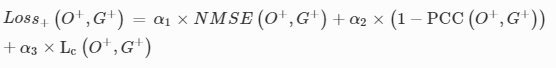## For the experimentally validated diffractive camera design shown in Fig. 7, an additional contrast loss Lc was added to Loss+ i.e.,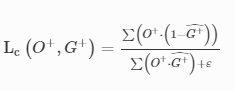## The coefficients (α1,α2,α3) were empirically set to (1, 3, 5) and Lc is defined as: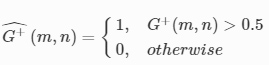## where ε=1e−6 was added to the denominator to avoid divide-by-zero error. G+ˆ is a binary mask indicating the transmissive regions of the input object G+, which is defined as:## By adding this image contrast related training loss term, the output images of the target objects exhibit enhanced contrast which is especially helpful in non-ideal experimental conditions. In addition, the MNIST training images were first linearly downsampled to 15 × 15 pixels and then upscaled to 90 × 90 pixels using nearest-neighbor interpolation. Then, the resulting input objects were augmented using the same parameters as described before and were fed into the diffractive camera for training. Each diffractive layer had 120 × 120 trainable diffractive neurons. To overcome the challenges posed by the fabrication inaccuracies and mechanical misalignments during the experimental validation of the diffractive camera, we vaccinated our diffractive model during the training by deliberately introducing random displacements to the diffractive layers . During the training process, a 3D displacement DD=(Dx,Dy,Dz) was randomly added to each diffractive layer following the uniform (U) random distribution: